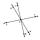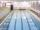# Angle of two lines

There is a regular quadrangular pyramid ABCDV; | AB | = 4 cm; height v = 6 cm. Determine the angles of lines AD and BV.

Result

X =  72.452 °

#### Solution:Leave us a comment of example and its solution (i.e. if it is still somewhat unclear...):Be the first to comment!## Next similar examples:

1. Clock faceclock face is given. Numbers 10 and 5, and 3 and 8 are connected by straight lines. Calculate the size of their angles.
2. The angle of linesCalculate the angle of two lines y=x-21 and y=-2x+14
3. PyramidPyramid has a base a = 5cm and height in v = 8 cm. a) calculate angle between plane ABV and base plane b) calculate angle between opposite side edges.
4. ConeThe rotating cone volume is 9.42 cm3, with a height 10 cm. What angle is between the side of the cone and its base?
5. MapleMaple peak is visible from a distance 3 m from the trunk from a height of 1.8 m at angle 62°. Determine the height of the maple.
6. ReflectorCircular reflector throws light cone with a vertex angle 49° and is on 33 m height tower. The axis of the light beam has with the axis of the tower angle 30°. What is the maximum length of the illuminated horizontal plane?
7. StairwayWhat angle rising stairway if step height in 17 cm and width 27 cm?
8. Reference angleFind the reference angle of each angle:On a straight stretch of road is marked 12 percent drop. What angle makes the direction of the road with the horizontal plane?
10. TreeHow tall is the tree that observed in the visual angle of 52°? If I stand 5 m from the tree and eyes are two meters above the ground.
11. High wallI have a wall 2m high. I need a 15 degree angle (upward) to second wall 4 meters away. How high must the second wall?
12. If theIf the tangent of an angle of a right angled triangle is 0.8. Then its longest side is. .. .Average climb of the road is given by ratio 1:15. By what angle road average climb?Road has climbing 1:27. How big is a angle corresponds to this climbing?Calculate slope (rise:run) of the bottom of swimming pool long 10 m. Water depth at beginning of pool is 1.16 m (for children) and depth at end is 1.89 m (for swimmers). Slope express as percentage and as angle in degrees.It is true that the lines that do not intersect are parallel?We want to prove the sentence: If the natural number n is divisible by six, then n is divisible by three. From what assumption we started?